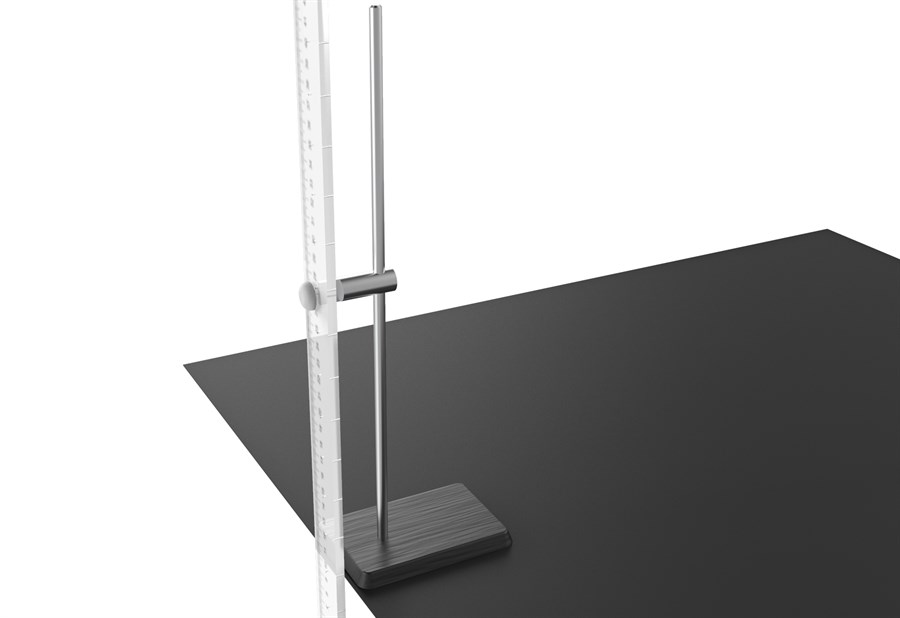# Swinging Rod

This simple swinging rod with a variable point of suspension allows one to investigate moment of inertia and the parallel axis theorem: a really simple , elegant and insightful mechanism to explain how the time period of an oscillator changes with moment of inertia about an axis. Use it to investigate nonlinear relationships and for connecting maths to physics.
Product Code: Swinging Rod
Brand: Qosain Scientific
Availability: In Stock

Rs. 10,000

The Experiment

• Mathematical model of a simple swinging rod.
• Investigating period, motion and moment of inertia.
• Application of the parallel axis theorem.
• Comparing theoretical predictions with exxperimental observation.
• Calculating uncertainities.

How does it work?

In this experiment, the object of interest is a rectangular wooden rod, specifically, a meter rule of mass M and length L. The rod can be hung on-to a pin pivoted on a firm post. To experiment with different moments of inertia, there is a series of holes drilled through the midline of the rod.

As shown in the figure, the position of the pin is P and the rod is made to swing in a vertical plane about this pivot. The center of mass is, of course, the geometric center of the wooden rod, point O. Students are required to measure the time period T of the oscillation with a stopwatch as the pivot P is varied and plot a graph between T and d = OP.

These observations are analysed for uncertainities and compared with the mathematical model.

Parts Included

• Stand
• Rod holder
• Rod
• Stopwatch
No Specifications Found